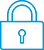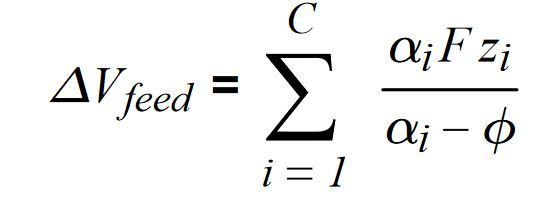cancel
Showing results for
Search instead for
Did you mean:It has been a while since anyone has replied. Simply ask a new question if you would like to start the discussion again.Level 1
10 3 0 0
Message 1 of 2
498
Flag Post

# Solve for variable in sumationHP Recommended
Hp prime
Microsoft Windows 10 (32-bit)

Hi, I would like to make a program that solves this equation for phi.

I have been attempting this all day but i keep getting errors.

EXPORT Vfeed(V_feed,F,q)

BEGIN

INPUT(M1);

INPUT(M2);

local s,eqn

s=0

FOR i=1:SIZE(M1)

eqn=M1(i)*F*M2(i)/(M1(i)-p)

end

solve(eqn=V_feed,p,1)

END;

given a  matrix for alphai and Zi. ( 1 column but n number of rows). I think it would be useful if my program could prompt the user for the number of rows and then the user inputs the to matrix. Then the program solves for phi.

I am having trouble with understanding how to index in order to solve this equation.Tags (4)
1 REPLY 1Level 6
305 297 32 76
Message 2 of 2
Flag PostHP Recommended

Hello Unluckyowl1,

I would suggest this program:

```EXPORT Vfeed(V_feed,F)
BEGIN
INPUT({{M1,},{M2,}});
LOCAL k;
L1:=SIZE(M1);
F1:=0;
FOR k FROM 1 TO L1(1) DO
F1:=F1+M1(k,1)*F*M2(k,1)/(M1(k,1)-'X');  //note the single quote signs around X in previous equatiom.
END;
F1:=F1-V_feed;
RETURN FNROOT(F1(X),X,1);

END;```

This program creates a function F1(X) which can be viewed in the function app which allows you to view all solutions of the equation, which are the intersections which the x-axis.

Never use a loop variable i, because i can not be the name of a variable, because it is a reserved name for the imaginary unit i, for which holds: i^2=-1.

Do not forget semicolumns!

Write := instead of =

For an automatic construction of a FOR loop:

In the menu of the Program editor press Tmplt - Loop - For.

Input of matrices with the INPUT command asks a special syntac.

4 is the TYPE of matrix variables. See the Help for INPUT.

You can not use an abstract variable p in a non-CAS program, therefore I made use of the predefined function F1(X) and wrote:

'X' between single quotes.

solve is a CAS command and cannot be used in this way in a non-CAS program.

Therefore I used the non-CAS command FNROOT.

Because SIZE of a matrix is a list with 2 elements I stored it into L1 and then used the first element for the loop.

The program assumes that M1 and M2 have the same number of rows.

When you have questions feel free to ask them.

† The opinions expressed above are the personal opinions of the authors, not of HP. By using this site, you accept the Terms of Use and Rules of Participation